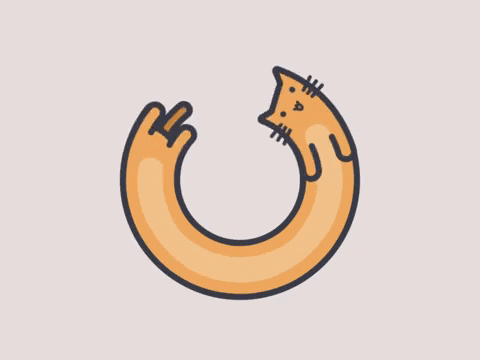Three.js 是一个 3D 库，该系列是在使用 three.js 中的一些过程和笔记。本文介绍物体的几何形状和材质等，并记录往场景中添加物体的基本过程。

# # 物体(Object)

## # 几何形状(Geometry)

WebGL 需要程序员指定每个顶点的位置，而在 Three.js 中，可以通过指定一些特征来创建几何形状。

Three 提供了立方体(其实是长方体)、平面(其实是长方形)、球体、圆形、圆柱、圆台等许多基本形状。 也可以通过自己定义每个点的位置来构造形状。 对于比较复杂的形状，我们还可以通过外部的模型文件导入。

## # 材质(Material)

• 基本材质(BasicMaterial)

• 使用基本材质(BasicMaterial)的物体，渲染后物体的颜色始终为该材质的颜色，而不会由于光照产生明暗、阴影效果。
• 如果没有指定材质的颜色，则颜色是随机的。
• Lambert 材质(MeshLambertMaterial)

• Lambert 材质(MeshLambertMaterial)是符合 Lambert 光照模型的材质
• Lambert 光照模型的主要特点是只考虑漫反射而不考虑镜面反射的效果，因而对于金属、镜子等需要镜面反射效果的物体就不适应，对于其他大部分物体的漫反射效果都是适用的
• Phong 材质(MeshPhongMaterial)

• Phong 材质(MeshPhongMaterial)是符合 Phong 光照模型的材质
• 和 Lambert 不同的是，Phong 模型考虑了镜面反射的效果，因此对于金属、镜面的表现尤为适合
• 法向材质(MeshNormalMaterial)

• 法向材质(MeshNormalMaterial)可以将材质的颜色设置为其法向量的方向，有时候对于调试很有帮助
• 材质的颜色与照相机与该物体的角度相关
• 材质的纹理贴图

• 使用图像作为材质，就需要导入图像作为纹理贴图，并添加到相应的材质中

# # 向场景中添加物体

## # 添加地板

``````// 添加地板
function initFloor() {
var floorGeo = new THREE.PlaneBufferGeometry(12, 8, 1, 1);
var floorMaterial = new THREE.MeshBasicMaterial({ color: "#aaaaaa" });
var floor = new THREE.Mesh(floorGeo, floorMaterial);
floor.position.set(0, 0, -1);
}
initFloor();
``````

## # 添加正方体

``````// 添加物体
function initCube(imageUrl) {
var geometry = new THREE.BoxGeometry(1, 1, 1);
var material;
if (imageUrl) {
material = new THREE.MeshLambertMaterial({
});
} else {
material = new THREE.MeshLambertMaterial();
}
var cube = new THREE.Mesh(geometry, material);
return cube;
}
``````

``````// 添加物体
var cube1 = initCube("./img/1.jpg");
var cube2 = initCube("./img/2.png");
var cube3 = initCube();
var cube4 = initCube();
cube1.position.set(2, 0, 0);
cube2.position.set(-2, 0, 0);
cube3.position.set(0, -2, 1);
cube4.position.set(1, 1, 3);
``````

## # 让物体动起来

``````// 让世界动起来
function render() {
requestAnimationFrame(render);

// 此处可添加动画处理
cube1.rotation.x += 0.03;
cube1.rotation.y += 0.03;

cube2.rotation.x += 0.02;
cube3.rotation.y += 0.01;
cube4.rotation.x -= 0.04;

renderer.render(scene, camera);
}
render();
``````

## # 完整代码

``````// 设置场景
var scene = new THREE.Scene();

// 创建正交投影照相机
var camera = new THREE.PerspectiveCamera(
75,
window.innerWidth / window.innerHeight,
0.1,
1000
);
camera.position.set(0, -5, 2);
camera.lookAt(scene.position);

// 定义着色器
var renderer = new THREE.WebGLRenderer();
renderer.setSize(window.innerWidth, window.innerHeight);
document.body.appendChild(renderer.domElement);

// 添加地板
function initFloor() {
var floorGeo = new THREE.PlaneBufferGeometry(12, 8, 1, 1);
var floorMaterial = new THREE.MeshBasicMaterial({ color: "#aaaaaa" });
var floor = new THREE.Mesh(floorGeo, floorMaterial);
floor.position.set(0, 0, -1);
}
initFloor();

// 添加物体
function initCube(imageUrl) {
var geometry = new THREE.BoxGeometry(1, 1, 1);
var material;
if (imageUrl) {
material = new THREE.MeshLambertMaterial({
});
} else {
material = new THREE.MeshLambertMaterial();
}
var cube = new THREE.Mesh(geometry, material);
return cube;
}
// 添加物体
var cube1 = initCube("./img/1.jpg");
var cube2 = initCube("./img/2.png");
var cube3 = initCube();
var cube4 = initCube();
cube1.position.set(2, 0, 0);
cube2.position.set(-2, 0, 0);
cube3.position.set(0, -2, 1);
cube4.position.set(1, 1, 3);

// 让世界动起来
function render() {
requestAnimationFrame(render);

// 此处可添加动画处理
cube1.rotation.x += 0.03;
cube1.rotation.y += 0.03;

cube2.rotation.x += 0.02;
cube3.rotation.y += 0.01;
cube4.rotation.x -= 0.04;

renderer.render(scene, camera);
}
render();
``````

# # 结束语